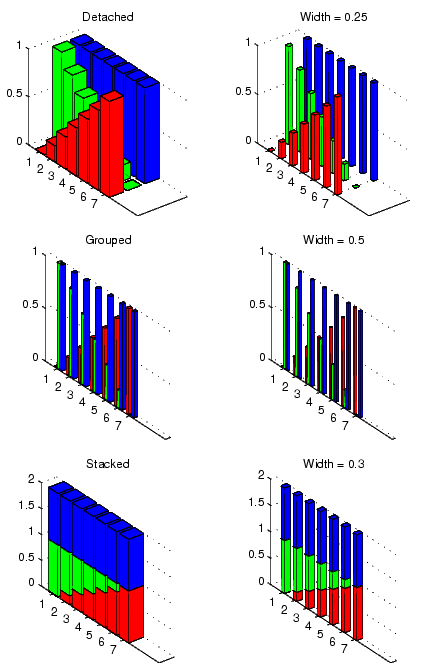MATLAB Function Referencebar3, bar3h

Plot three-dimensional bar chart

Syntax

• ```bar3(Y)
bar3(x,Y)
bar3(...,width)
bar3(...,'`style`')
bar3(...,`LineSpec`)
bar3(axes_handle,...)
h = bar3(...)

bar3h(...)
h = bar3h(...)
```

Description

`bar3` and `bar3h` draw three-dimensional vertical and horizontal bar charts.

```bar3(Y) ``` draws a three-dimensional bar chart, where each element in `Y` corresponds to one bar. When `Y` is a vector, the x-axis scale ranges from `1` to `length(Y)`. When `Y` is a matrix, the x-axis scale ranges from `1` to `size(Y,2)`, which is the number of columns, and the elements in each row are grouped together.

```bar3(x,Y) ``` draws a bar chart of the elements in `Y` at the locations specified in `x`, where `x` is a vector defining the y-axis intervals for vertical bars. The x-values can be nonmonotonic, but cannot contain duplicate values. If `Y` is a matrix, `bar3` clusters elements from the same row in `Y` at locations corresponding to an element in `x`. Values of elements in each row are grouped together.

```bar3(...,width) ``` sets the width of the bars and controls the separation of bars within a group. The default `width` is `0.8`, so if you do not specify `x`, bars within a group have a slight separation. If `width` is `1`, the bars within a group touch one another.

```bar3(...,'style') ``` specifies the style of the bars. `'``style``' `is `'detached'`, `'grouped'`, or `'stacked'`. `'detached'` is the default mode of display.

• `'detached'` displays the elements of each row in `Y` as separate blocks behind one another in the x direction.
• `'grouped'` displays n groups of m vertical bars, where n is the number of rows and m is the number of columns in `Y`. The group contains one bar per column in `Y`.
• `'stacked'` displays one bar for each row in `Y`. The bar height is the sum of the elements in the row. Each bar is multicolored, with colors corresponding to distinct elements and showing the relative contribution each row element makes to the total sum.

```bar3(...,LineSpec) ``` displays all bars using the color specified by `LineSpec`.

```bar3(axes_handles,...) ``` plots into the axes with handle `axes_handle` instead of the current axes (`gca`).

```h = bar3(...) ``` returns a vector of handles to patch graphics objects. `bar3` creates one patch object per column in `Y`.

```bar3h(...) and h = bar3h(...) ``` create horizontal bars. `Y` determines the bar length. The vector `x` is a vector defining the y-axis intervals for horizontal bars.

Examples

This example creates six subplots showing the effects of different arguments for `bar3`. The data `Y` is a seven-by-three matrix generated using the `cool` colormap:

• ```Y = cool(7);
subplot(3,2,1)
bar3(Y,'detached')
title('Detached')
subplot(3,2,2)
bar3(Y,0.25,'detached')
title('Width = 0.25')
subplot(3,2,3)
bar3(Y,'grouped')
title('Grouped')
subplot(3,2,4)
bar3(Y,0.5,'grouped')
title('Width = 0.5')
subplot(3,2,5)
bar3(Y,'stacked')
title('Stacked')
subplot(3,2,6)
bar3(Y,0.3,'stacked')
title('Width = 0.3')
colormap([1 0 0;0 1 0;0 0 1])```

See Also

`bar`, `LineSpec`, `patch`

Area, Bar, and Pie Plots for related functions

Bar and Area Graphs for more examplesbar, barh Barseries Properties© 1994-2005 The MathWorks, Inc.